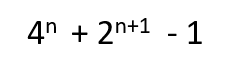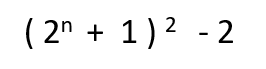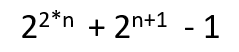GeeksforGeeks App
Open AppBrowser
Continue

# Program to find the nth Kynea number

Given a positive integer n, the task is to find nth Kynea number.
Kynea number: In mathematics, a Kynea number is a positive integer of the form:where n is a positive integer.
The equivalent formula for nth Kynea number is:The first few Kynea number are:

7, 23, 79, 287, 1087, 4223, 16639, 66047, 263167, 1050623, 4198399, …..

Examples:

Input: 2
Output: 23
Putting n = 2 in formula,
= 42 + 2 2+1 – 1
= 16 + 8 -1
= 23

Method 1: A Simple Solution is to find out the nth number by putting the value of n in the formulaBelow is the implementation of the above approach:

## C++

 `// CPP code to find nth Kynea number` `#include ``using` `namespace` `std;` `// Function to calculate nth kynea number``long` `nthKyneaNumber(``int` `n)``{` `    ``// Calculate nth kynea number``    ``// using formula ((2^n + 1)^2 ) -2` `    ``// Firstly calculate 2^n + 1``    ``n = (1 << n) + 1;` `    ``// Now calculate (2^n + 1)^2``    ``n = n * n;` `    ``// Now calculate ((2^n + 1)^2 ) - 2``    ``n = n - 2;` `    ``// return nth Kynea number``    ``return` `n;``}` `// Driver Program``int` `main()``{``    ``int` `n = 8;` `    ``// print nth kynea number``    ``cout << nthKyneaNumber(n);` `    ``return` `0;``}`

## Java

 `// JAVA code to find nth Kynea number` `class` `GFG {` `    ``// Function to calculate nth kynea number``    ``static` `long` `nthKyneaNumber(``int` `n)``    ``{` `        ``// Calculate nth kynea number``        ``// using formula ((2^n + 1)^2 ) -2` `        ``// Firstly calculate 2^n + 1``        ``n = (``1` `<< n) + ``1``;` `        ``// Now calculate (2^n + 1)^2``        ``n = n * n;` `        ``// Now calculate ((2^n + 1)^2 ) - 2``        ``n = n - ``2``;` `        ``// return nth Kynea number``        ``return` `n;``    ``}` `    ``// Driver Program``    ``public` `static` `void` `main(String[] args)``    ``{``        ``int` `n = ``2``;` `        ``// print nth kynea number``        ``System.out.println(nthKyneaNumber(n));``    ``}``}`

## Python

 `# Python code to find nth Kynea number` `# Function to calculate nth kynea number``def` `nthKyneaNumber( n):``    ` `    ``# Calculate nth kynea number``    ``# using formula ((2 ^ n + 1)^2 ) -2``    ` `    ``# Firstly calculate 2 ^ n + 1``    ``n ``=` `( ``1` `<< n) ``+` `1``    ` `    ``# Now calculate (2 ^ n + 1)^2``    ``n ``=` `n ``*` `n``    ` `    ``# Now calculate ((2 ^ n + 1)^2 ) - 2``    ``n ``=` `n``-``2``    ` `    ` `    ``# return nth Kynea number``    ``return` `n``    `   `# Driver Code``n ``=` `2` `# print nth kynea number``print``(nthKyneaNumber(n))`

## C#

 `// C# code to find nth Kynea number` `using` `System;``class` `GFG {` `    ``// Function to calculate nth kynea number``    ``static` `long` `nthKyneaNumber(``int` `n)``    ``{` `        ``// Calculate nth kynea number``        ``// using formula ((2^n + 1)^2 ) -2` `        ``// Firstly calculate 2^n + 1``        ``n = (1 << n) + 1;` `        ``// Now calculate (2^n + 1)^2``        ``n = n * n;` `        ``// Now calculate ((2^n + 1)^2 ) - 2``        ``n = n - 2;` `        ``// return nth Kynea number``        ``return` `n;``    ``}` `    ``// Driver Program``    ``public` `static` `void` `Main()``    ``{``        ``int` `n = 2;` `        ``// print nth kynea number``        ``Console.WriteLine(nthKyneaNumber(n));``    ``}``}`

## PHP

 `

## Javascript

 ``

Output:

`66047`

Time Complexity: O(1), only constant operations are being used.
Auxiliary Space: O(1), as no extra space is required.

Method 2: This solution is based on the fact that every Kynea number follows a specific pattern in their binary representation. nth Kynea number can be represented in binary as a single leading one followed by exactly n-1 consecutive 0’s, followed by n+1 consecutive 1’s.

Example:

```23 is 2nd kynea number
It can be represented in binary as 10111
(Single leading one, followed by n - 1 ( i.e 2-1=1 ) consecutive 0's,
followed by n + 1 ( i.e 2 + 1 = 3 ) consecutive 1's.)```

Observing the binary pattern of Kynea number in above table, the nth Kynea number can be easily calculated using the formula:Example:

Input: n = 3
Output: 79
Using formula,
= 26 + 24 -1
= 64 + 15
= 79

Below is the implementation of the above approach

## C++

 `// CPP code to find nth Kynea number` `#include ``using` `namespace` `std;` `// Function to calculate nth kynea number``long` `nthKyneaNumber(``int` `n)``{` `    ``// Calculate nth kynea number``    ``return` `((1 << (2 * n)) + (1 << (n + 1)) - 1);``}` `// Driver Program``int` `main()``{``    ``int` `n = 2;` `    ``// print nth kynea number``    ``cout << nthKyneaNumber(n);` `    ``return` `0;``}`

## Java

 `// JAVA code to find nth Kynea number` `class` `GFG {` `    ``// Function to calculate nth kynea number``    ``static` `long` `nthKyneaNumber(``int` `n)``    ``{` `        ``// Calculate nth kynea number``        ``return` `((``1` `<< (``2` `* n)) + (``1` `<< (n + ``1``)) - ``1``);``    ``}` `    ``// Driver Program``    ``public` `static` `void` `main(String[] args)``    ``{``        ``int` `n = ``2``;` `        ``// print nth kynea number``        ``System.out.println(nthKyneaNumber(n));``    ``}``}`

## Python

 `# Python code to find nth Kynea number` `# Function to calculate nth kynea number``def` `nthKyneaNumber( n):` `    ``# Calculate nth kynea number   ``    ``return` `(( ``1` `<< (``2` `*` `n)) ``+` `( ``1` `<< (n ``+` `1``)) ``-``1` `)``    `  `# Driver Code``n ``=` `2` `# print nth kynea number``print``(nthKyneaNumber(n))`

## C#

 `// C# code to find nth Kynea number` `using` `System;``class` `GFG {` `    ``// Function to calculate nth kynea number``    ``static` `long` `nthKyneaNumber(``int` `n)``    ``{` `        ``// Calculate nth kynea number``        ``return` `((1 << (2 * n)) + (1 << (n + 1)) - 1);``    ``}` `    ``// Driver Program``    ``public` `static` `void` `Main()``    ``{``        ``int` `n = 2;` `        ``// print nth kynea number``        ``Console.WriteLine(nthKyneaNumber(n));``    ``}``}`

## PHP

 ``

## Javascript

 ``

Output:

`23`

Time Complexity: O(1), only constant operations are being used.
Auxiliary Space: O(1), as no extra space is required.

My Personal Notes arrow_drop_up